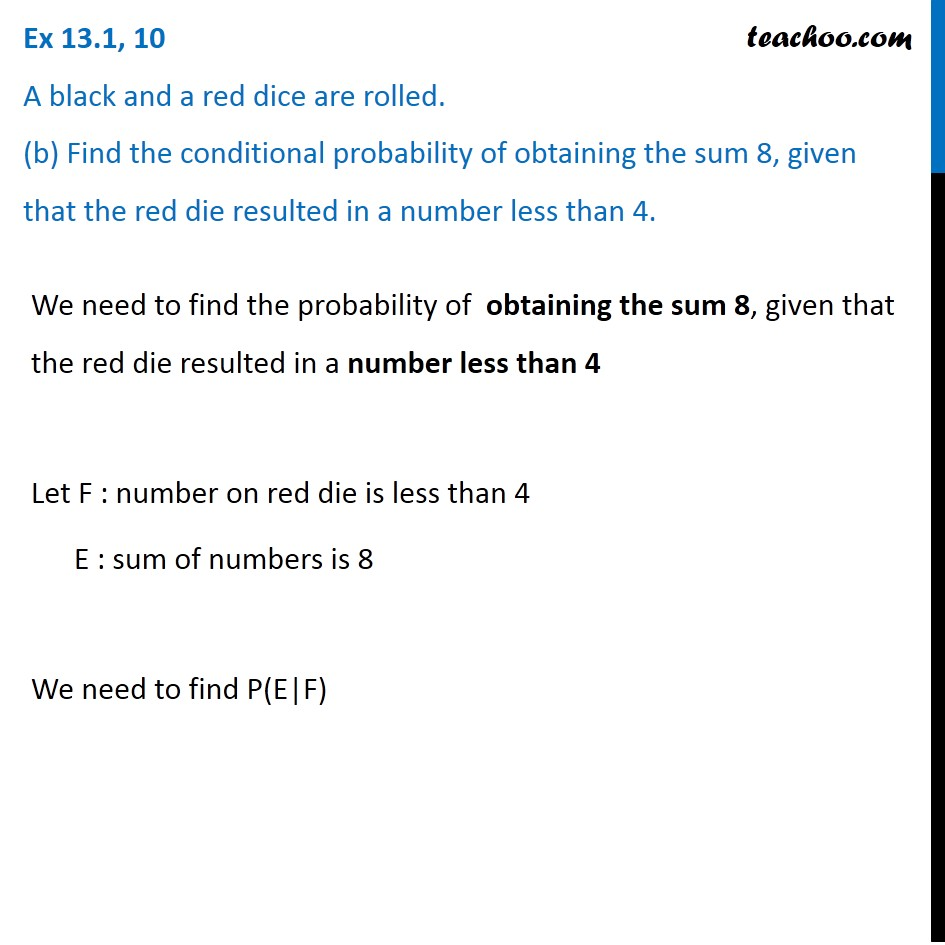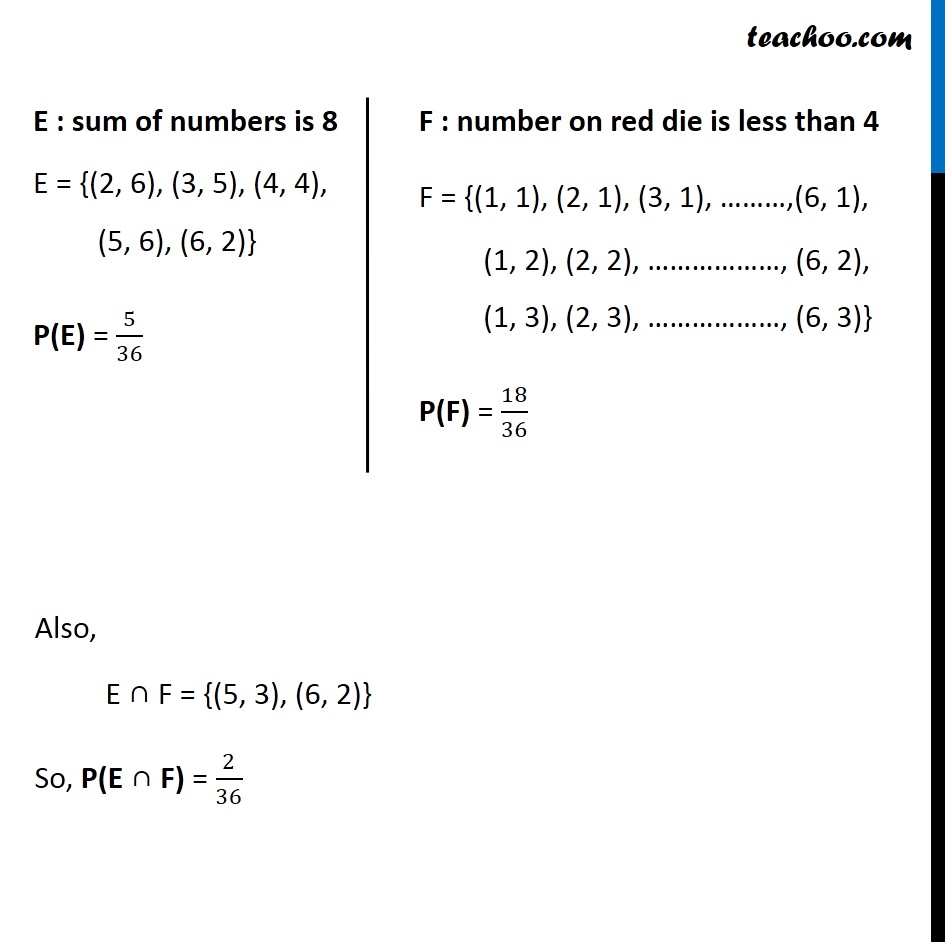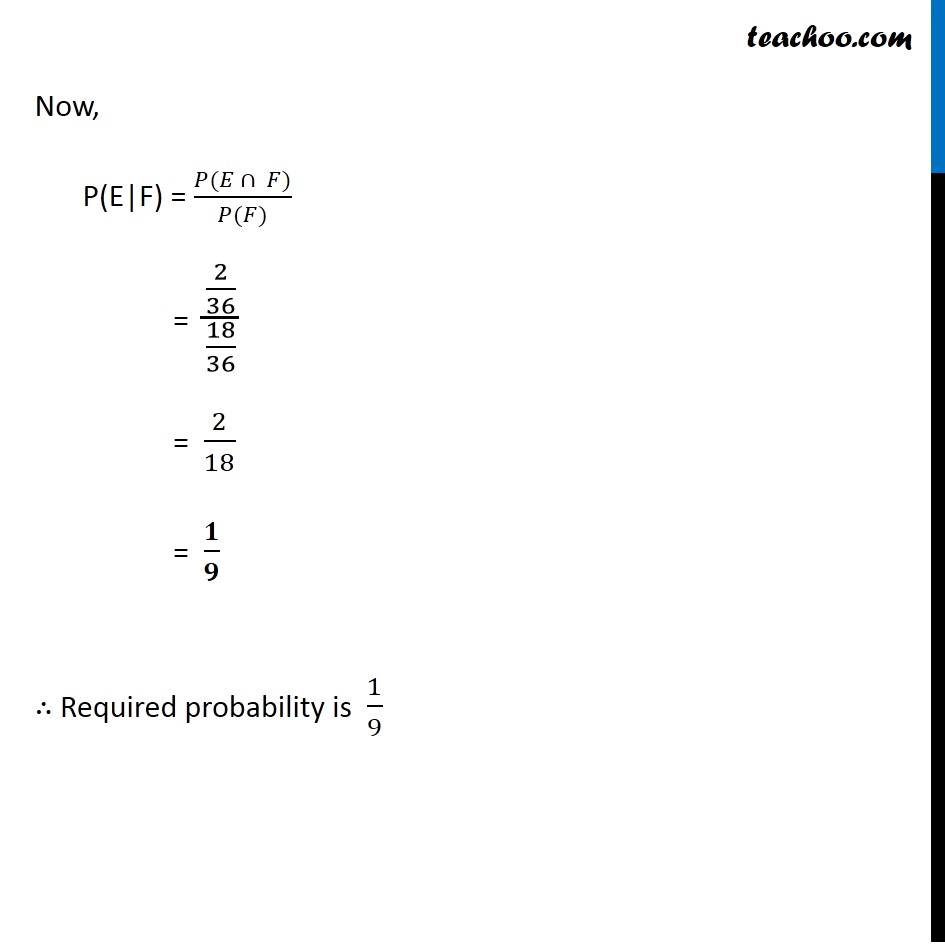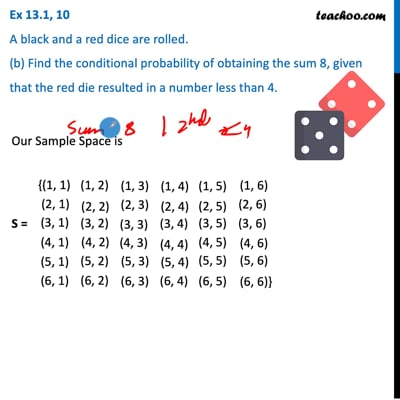Ex 13.1

Chapter 13 Class 12 Probability
Serial order wiseThis video is only available for Teachoo black users

Maths Crash Course - Live lectures + all videos + Real time Doubt solving!

### Transcript

Ex 13.1, 10 A black and a red dice are rolled. (b) Find the conditional probability of obtaining the sum 8, given that the red die resulted in a number less than 4.We need to find the probability of obtaining the sum 8, given that the red die resulted in a number less than 4 Let F : number on red die is less than 4 E : sum of numbers is 8 We need to find P(E|F) E : sum of numbers is 8 E = {(2, 6), (3, 5), (4, 4), (5, 6), (6, 2)} P(E) = 5/36 F : number on red die is less than 4 F = {(1, 1), (2, 1), (3, 1), ………,(6, 1), (1, 2), (2, 2), ………………, (6, 2), (1, 3), (2, 3), ………………, (6, 3)} P(F) = 18/36 Also, E ∩ F = {(5, 3), (6, 2)} So, P(E ∩ F) = 2/36 Now, P(E|F) = (𝑃(𝐸 ∩ 𝐹))/(𝑃(𝐹)) = (2/36)/(18/36) = 2/18 = 𝟏/𝟗